# How to Remove Middle Name from Full Name in Excel

When we get a list of full names, we can remove the middle name for short in some cases. As the middle names are different among the full name list so we cannot replace them by space directly in excel. This article will provide you two methods to remove the middle name. User can use Formula to remove the middle name, or can remove it by Find and Replace function.

Prepare a list of full names.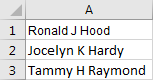## Remove the Middle Name from Full Name by Formula

Step1: Select a blank cell next to the full name, for example select B1. Enter the following formula into B1:

=TRIM(LEFT(A1,FIND(” “,LOWER(A1),1))) & ” ” & TRIM(MID(A1,FIND(” “,LOWER(A1),FIND(” “,LOWER(A1),1)+1)+1,LEN(A1)-FIND(” “,LOWER(A1),1)+1)) .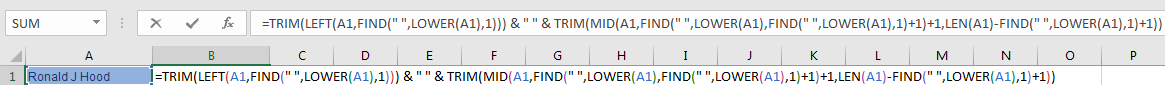Step2: Click Enter and get the result.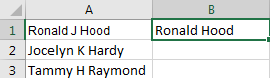Step3: Drag the Auto Fill handle to fill B2 and B3. Now all the middle names are removed from the list.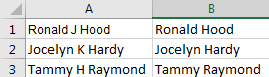## Remove the Middle Name from Full Name by Find and Replace Function

Step1: Click Ctrl+F to load Find and Replace window.

Step2: Under Replace tab, in Find what textbox enter ‘ * ‘ (a space + * + a space); in Replace with textbox enter ‘ ‘ (a space). Then click Replace All.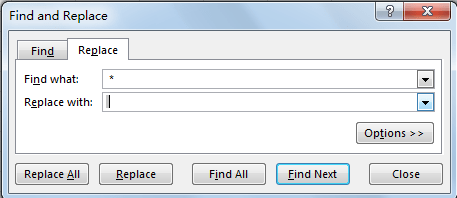Step3: Check the result. All the middle names are removed.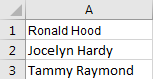### Related Functions

• Excel LEFT function
The Excel LEFT function returns a substring (a specified number of the characters) from a text string, starting from the leftmost character.The LEFT function is a build-in function in Microsoft Excel and it is categorized as a Text Function.The syntax of the LEFT function is as below:= LEFT(text,[num_chars])…t)…
• Excel FIND function
The Excel FIND function returns the position of the first text string (sub string) within another text string.The syntax of the FIND function is as below:= FIND(find_text, within_text,[start_num])…
• Excel TRIM function
The Excel TRIM function removes all spaces from text string except for single spaces between words.  You can use the TRIM function to remove extra spaces between words in a string.The syntax of the TRIM function is as below:= TRIM (text)….
• Excel LEN function
The Excel LEN function returns the length of a text string (the number of characters in a text string).The syntax of the LEN function is as below:= LEN(text)…
• Excel LOWER function
The Excel LOWER function converts all characters in a text string to lowercase.The syntax of the LOWER function is as below:= LOWER  (text)…

Sidebar